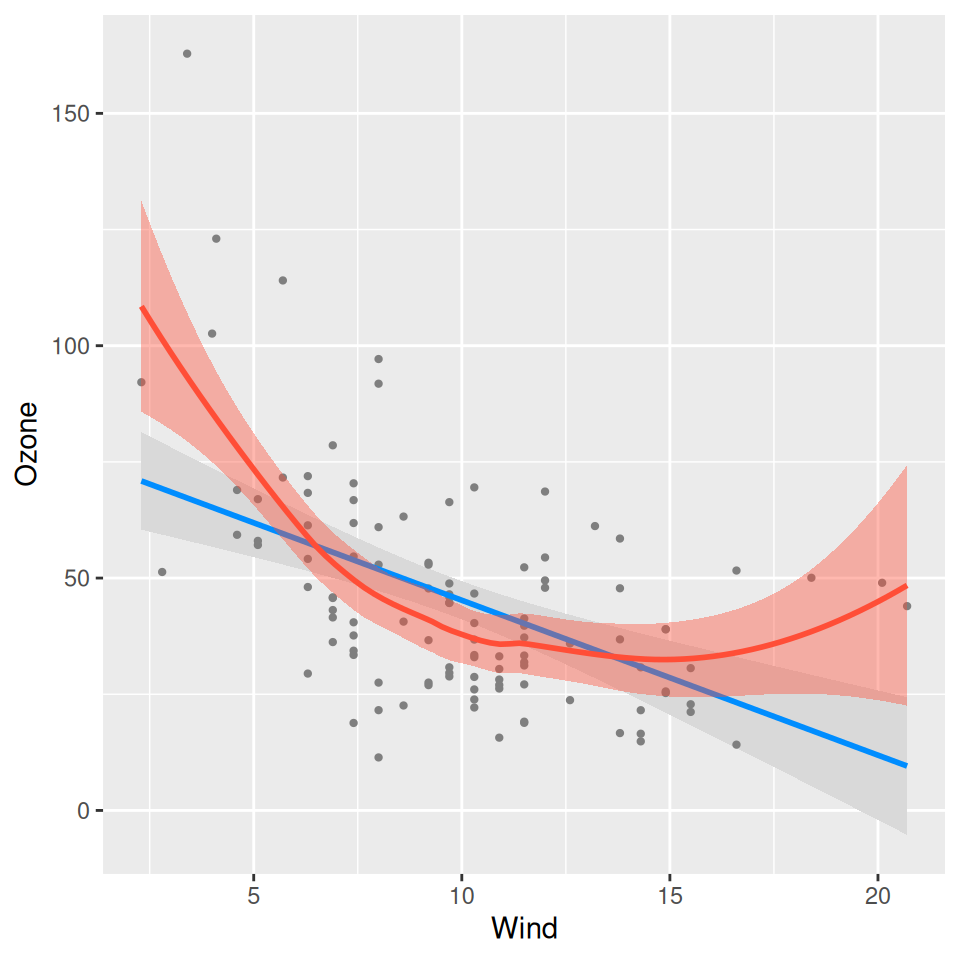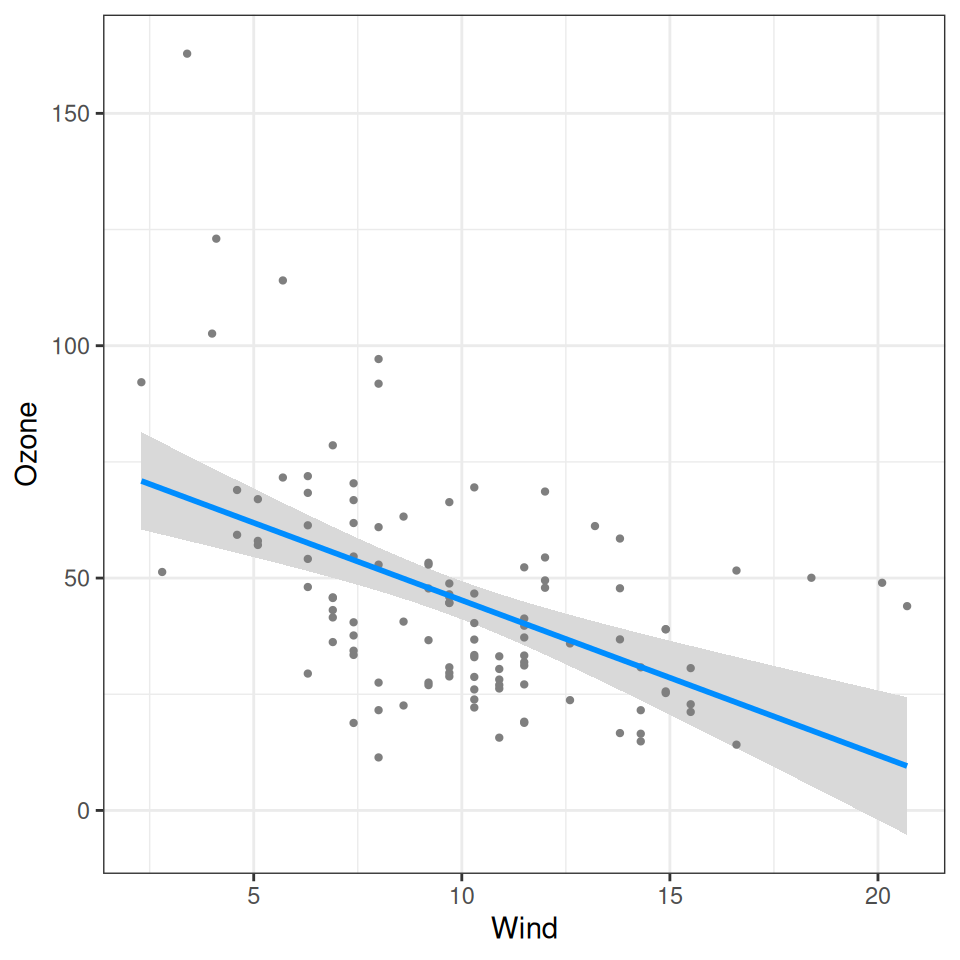By default, visreg will use base R graphics as the engine; however, you also have the option of using ggplot2 as the engine. For example:

fit <- lm(Ozone ~ Solar.R + Wind + Temp, data=airquality)
visreg(fit, "Wind", gg=TRUE)Graphical options regarding the appearance of points, lines, and bands are specified in the same way as with base R:

visreg(fit, "Wind", gg=TRUE, line=list(col="red"),
fill=list(fill="green"),
points=list(size=5, pch=1))Note that visreg returns a gg object, and therefore, you can use ggplot2 to add additional layers to the graph. For example, we could add a smoother:

visreg(fit, "Wind", gg=TRUE) + geom_smooth(method="loess", col='#FF4E37', fill='#FF4E37')
# geom_smooth() using formula 'y ~ x'Or we could modify the theme:

visreg(fit, "Wind", gg=TRUE) + theme_bw()